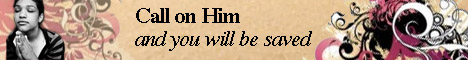Note:  Do not rely on this information. It is very old.

# Quadrature, Methods of

Quadrature, Methods of. When the surface whose area is required is bounded by straight lines, the area can be found simply by splitting it up into triangles. The areas of segments of some curves are also easily found; for example, the area of a parabolic segment is equal to two-thirds that of the triangle whose sides are the chord of the segment and the tangents at its extremities, or four-thirds that of the triangle on the same base, but whose vertex is the point on the curve cut by the diameter, through the point of intersection of the tangents. For any curve the following method is applicable:- Divide the base into a number of equal parts b, and erect ordinates at the points of division. Let ordinates (represented by the dotted lines) also be erected half-way between these others, their heights being h1, h2, h3 ... The product bh1 represents the area formed by the figure on base b, whose sides are the two first ordinates produced to meet the fourth side, the tangent at the top of h1. Hence the area of the figure A B C D is rather less than b (h1 + h2 + h3 + ... hn) but this expression becomes nearer the true value the smaller we take b. If b is so small that it can be written dx, and the ordinates are different values of y, the expression for the area may be written fydx between the limits y = AB and y = DC. In some cases this integral cannot be definitely determined, but different methods of approximation can be found. In Simpson's method for determining areas, small parts of the curve are considered as arcs of a parabola, whose axis is taken parallel to the ordinates, the base being divided into an even number of equal parts. A rough approximation to the area of any surface may be obtained by cutting the surface out in uniformly thick paper, weighing it, and comparing it with the weight of a known area.